Reach Us+44 1704 335730
Calculation of Critical Distance in Faulted Meshed Power System | OMICS International
Global Journal of Technology and Optimization
All submissions of the EM system will be redirected to Online Manuscript Submission System. Authors are requested to submit articles directly to Online Manuscript Submission System of respective journal.

# Calculation of Critical Distance in Faulted Meshed Power System

Hedaya Alasooly*

University of Swaziland, Swaziland

*Corresponding Author:
Hedaya Alasooly
University of Swaziland, Swaziland
E-mail: [email protected]

Received date: March 2011; Revised date: July 2011; Accepted date: September 2011

Visit for more related articles at Global Journal of Technology and Optimization

#### Abstract

Faults studies form an important part of power system analysis. The problem consists of determining bus voltages and line currents during various types of faults. If the fault location is known, the problem is easy to solve. But if the fault location is unknown, the problem will become more complex. The problem of fault location has been studied deeply for transmission lines due its importance in the power system. Different methods for sags prediction have been developed. The most used are “critical distance” and “fault positions”. The critical distance method is based on the concept of potential divider, which is correctly and easily applicable to a radial network. The extension of this method to large meshed networks has been discussed but yet non of the existing researches could provide proper solution for the problem. In this paper, an elegant, analytical method is developed to calculate the critical distance of a three-phase fault on transmission line that will cause certain voltage dip at a bus in meshed power system. The method is based in Gauss-Seidel iteration. The proposed method is tested on 6-bus transmission network and the results showed significant advantages of the proposed method .

#### Keywords

Fault analysis, meshed power system, three-phase balanced fault, voltage dip, Gauss-Seidel, load flow, critical distance.

#### Introduction

Faults studies form an important part of power system analysis. The problem consists of determining bus voltages and line currents during various types of faults. Faults on power system are divided into three phase balanced fault and unbalanced faults [1,2,3]. Different types of unbalanced faults are single line to ground fault, line-to-line fault, and double line to ground fault [1,2,3]. The information gained from fault studies are used for proper relay setting and coordination. The three-phase balanced fault information is used to select and set phase relays, while line to ground fault is used for ground relays. Fault studies are also used to obtain the rating of the protective switchgears.

The power system faults studies and analysis have been covered in many references. ,  and  are good references in this area. All this analysis are based on known location of the fault, so the problem was easy to solve.

Nowadays the problem of fault location on distribution systems is receiving special attention mainly because of the power quality regulations. Different methods for sags prediction have been developed. The most used are “critical distance” and “fault positions”. The critical distance method is based on the concept of potential divider, which is correctly and easily applicable to a radial network. The extension of this method to large meshed networks has been discussed in [4,5], but questionable assumptions are required. So, the applicability of the critical distance method to meshed networks is limited to very preliminary results only.

For meshed networks, the method of fault positions is usually adopted [4,6]. It requires modelling the electrical net and simulating faults in different positions. However, there are no clear and general rules to determine the part of the network to be analyzed and the positions and number of the faults to be simulated. An elegant, analytical method to predict voltage sags caused by three-phase faults on transmission networks has been developed in . Voltage sags at the given site are calculated through the bus impedance matrix of the net. The voltage sags prediction is not made through the individuation of the exposed areas, but through the probability density functions of voltage sags. However, this method is far less “spontaneous” than the method of fault positions, and its application is more complex.  introduced a method for obtaining a first estimate of the expected number of spurious trips due to voltage sags. The method is based on an expression for the so-called critical distance in radial power systems.  derived analytical expressions for the calculation of voltage sag magnitude due to faults at every point of a meshed or radial power network considering balanced and unbalanced faults. The following methods for stochastic assessment of voltage sag magnitude are compared using these expressions: the method of critical distances, method of fault positions, and Monte Carlo method.  presented some of the most relevant methods for fault location in radial power systems.

The objective of this paper is to propose an efficient method to solve the critical distance problem when there is a three-phase fault on transmission line in meshed power system. The method is based on Gauss-Seidel iteration.

The paper starts by giving a brief description to the solution of the balanced three-phase to ground short circuit fault problem and the power flow solution using Gauss-Seidel. Then, the proposed approach for the calculation of critical distance in meshed power system is introduced. The proposed method is tested on 6-bus transmission network and the results showed significant advantages of the proposed method.

#### Balanced three phases to ground short circuit fault analysis

In this paper, the balanced three phases to ground short circuit fault on transmission system is studied. The magnitude of fault current depends on the internal impedance of the generator plus the impedance of intervening circuit. The bus impedance matrix is formulated for the systematic computation of bus voltages and line currents during the fault.

 and  introduced the application of Thevenin‟s theorem in power system fault analysis. The fault is simulated by switching on fault impedance Zf at the faulted bus. Thevenin‟s theorem states that the changes in the network voltage caused by added branch (the fault impedance) are equivalent to those caused by the added voltage at faulted bus (0) Vf with all other sources short circuited.

So, the faulted network is reduced into Thevenin„s equivalent circuit as viewed from the faulted bus. Applying Thevenin„s theorem, changes in the bus voltages are obtained. Bus voltages are obtained by superposition of the pre-fault bus voltage and the changes in the bus voltages computed. The currents during the fault in all branches of the network are then obtained.

The network reduction is not efficient and not applicable to large system . Consider a typical general n bus system in Figure 1 . The system is assumed to be operating under balanced condition and per phase circuit model is used. Each machine is represented by constant voltage source behind proper reactance which may be X''d ,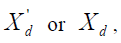where Xd is the synchronous machine direct axis reactance, and X''d is the synchronous machine subtransient direct axis reactance. The transmission lines are represented by equivalent π model and all impedances are expressed in per unit on a common Volt Ampere (VA) base. A balanced three-phase fault is to be applied at the bus k through a fault impedance Zf.

The pre-fault bus voltages are obtained from the power flow solution and are represented by the column vector

The short circuit currents are so much larger than the steady state current values, so that the latter can be neglected. However, a good approximation is to represent the bus load by constant voltage evaluated at the pre fault bus voltage

Where,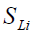and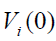are the apparent power and the pre-fault voltage at bus i respectively. The changes in the network voltage caused by the fault with impedance Zf. are equivalent to those caused by the added voltage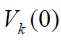with the other sources short circuited. Zeroing all voltage sources and representing all components by their impedances, the Thevenin„s circuit is obtained. The bus voltage changes caused by the fault in the circuit are represented by the column vector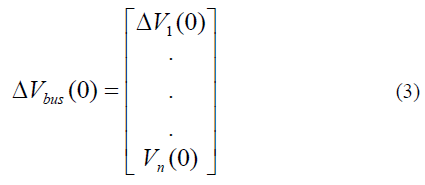From the Thevenin„s theorem, the bus voltages of system buses during the fault are obtained by superposition of the pre-fault bus voltages and the changes in the bus voltages given by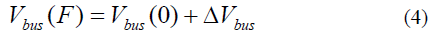In the node-voltage equation for the n-bus network, the injected bus currents are expressed in term of bus voltages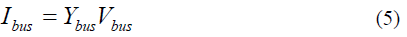In the Thevenin„s circuit shown in Figure 1, current entering every bus is zero except at the faulted bus. Since the current at faulted bus is leaving the bus, it is taken as a negative current entering bus k . Thus, the nodal equation applied to the Thevenin„s circuit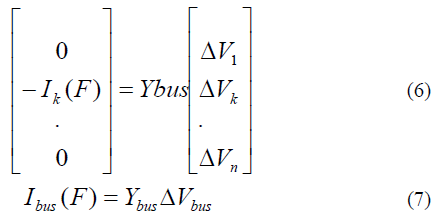Solving for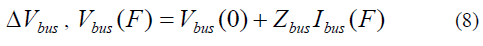Since there is one single nonzero element in the system, the kth equation becomes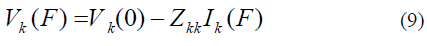Also from the Thevenin„s circuit shown in Figure 1,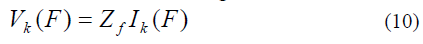For bolted fault,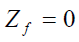and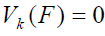. Substituting for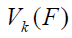from (10) into (9) and solving for the fault current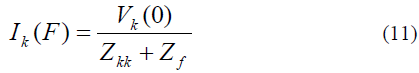Thus, for the fault at bus k, only the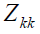element of the bus impedance matrix is needed. This element is indeed the Thevenin„s impedance as viewed from the faulted bus. Also, writing the ith equation in (8)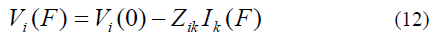Substituting for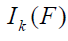, bus voltage during fault at bus i becomes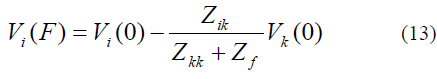With the knowledge of bus voltages during the fault, the fault current in all lines can be calculated. For the line connecting buses i and j with the impedance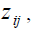the short circuit current in this line is defined by (14)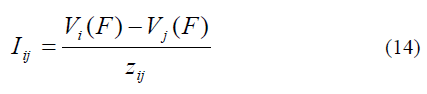This analysis assumed that the fault location is known, and so the problem was easy to solve. But if the fault location is unknown, the problem will become more complex.

#### Gauss-Seidel power flow solution

The power flow solution using Gauss-Seidel has been covered in many references. Detailed Gauss-Seidel power flow analysis can be found in ,  and .

The Gauss-Seidel method is known as the method of sucessive displacements. Consider the solution of nonlinear equation given by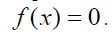The above equation is rearranged and written as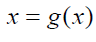(15)

If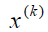is an intial estimate for the variable x , the following iterative sequence is performed.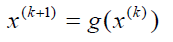(16)

A solution is obtained when the difference between the absulate value of the successive iteration is less than a specified accuracy, i.e.,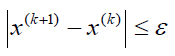(17)

In solving a power flow problem, the system is assumed to be operating under balanced conditions and a single-phase model is used. Four quantities are associated with each bus. These are voltage magnitude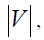phase angle θ , real power P and reactive power Q . The system buses are generally classified into three types: slack bus, load bus or voltage controlled bus [1,2,3].

The slack bus is a bus where the magnitude and phase angle of the voltage is specified. The load bus (PQ bus) is a bus where the active and reactive powers are specified while the magnitude and the phase angle of the bus voltage are unknown. The voltage controlled bus (PV bus) is a bus where the real power and voltage magnitude are specified while the phase angle of voltage and reactive power to be determined. The limits on the value of the reactive power is also specified.

Consider a typical bus of a power system as

shown in Figure 2. Transmission lines are represented by their equivaent π models where impedances have been converted to per unit admittances on common Volt Ampere (VA) base.

Application of KCL to this bus results in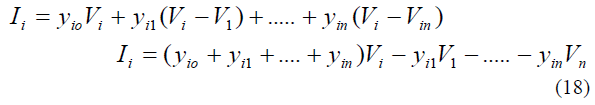The real and reactive power in bus i is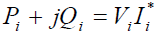(19)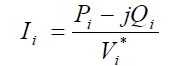(20)

Substituting for Ii in (18) yields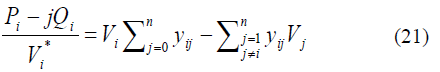In the power flow study, it is necessary to solve the set of nonlinear equations represented by (21) for two unknown variables at each node. In the Gauss-seidel method, (21) is solved for Vi and the iterative sequence becomes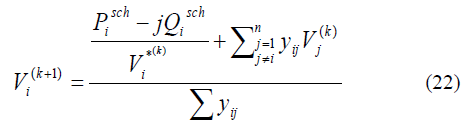The power flow equation is usually expressed in terms of the elements of bus admittance, then (22) becomes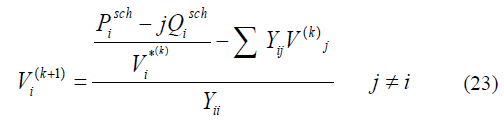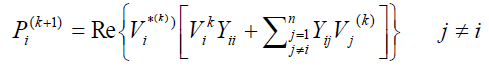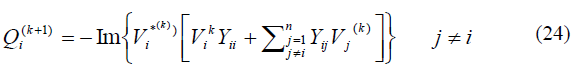Since the voltage is known at any slack system in the system, the above equation (23) must be solved for all other unknown node voltages each iteration.

An initial estimate of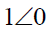for unknown voltages is satisfactory. For PQ buses, the real and reactive powers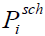and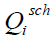are known. Starting with initial estimate, (23) is solved for the voltage. For the voltage controlled buses (PV buses), whereand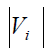are specified, first (24) is solved for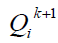, and then is used in in (23) to solve for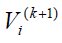. However, since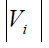is specified, only the angle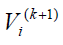of is retained.

The updated voltages immediately replace the previous values in the solution of subsequent equations. The process is contined until the changes of bus voltages between sucessive iterations are within satesfactory accuracy, i.e.,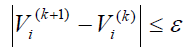(25)

#### The proposed procedure for the solution of critical distance problem in meshed power system

The proposed procedure for the solution of critical distance problem in meshed power system can be explained through the following simple network shown in Figure 3.

The procedure can be summarized as followed:

STEP 1. Add a node at the point in the line at which the fault will occur, in our example the fault is assumed to occur at node 3 in the line between node 2 and node 4. So, the problem is to find the fault location in the line by calculating the impedance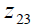that will cause certain voltage dip at a bus, i.e. bus 2, given total line impedance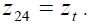STEP 2. Assume initially that the fault is existing at middle of the line, so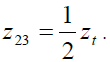STEP 3. Calculate the system Ybus matrix, and the positive sequence impedance matrix of the system which can be found by zeroing all voltage sources and representing all components with their impedances.

STEP 4. Calculate the all bus voltages using the previously mentioned fault analysis procedure assuming a three-phase fault at node 3.

STEP 5. Now, consider each generator bus as slack bus, i.e. bus 4 and bus 5, with its voltage magnitude equal to the value obtained from the fault analysis solution. Consider bus 2 as a slack bus with its voltage magnitude equal to the desired voltage dip magnitude, ie. V2=0.2 pu, and the faulted bus 3 as a slack bus with its voltage magnitude specified by the value obtained from the fault analysis solution. Use the Gauss-Seidel iterative method  to obtain the solution for the unknown bus voltage V4 and the line impedance z23 that will cause the given amount of voltage dip at bus 2, ie. V2=0.2 pu. The following equations should be used in the Gauss- Seidel iteration. The node equation at node 2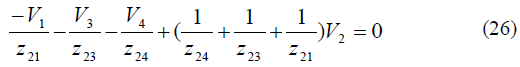Thus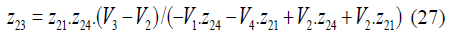The node equation at node 4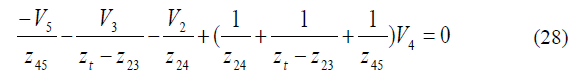Rearranging,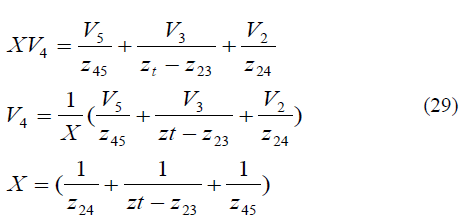STEP 6. Repeat steps 3 and 4 to solve the fault analysis problem and calculate the fault voltage at all system buses with the values ofand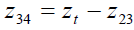obtained in the previous step.

STEP 7. Repeat step 5 to obtain the Gauss-Seidel solution forand V4 with other buses are considered as slack buses with the voltage magnitudes specified as mentioned in step 5.

STEP 8. Repeat steps 3, 4 and 5 with the updated variables replace the previous values in the solution of subsequent equations. The process is contined until the final solution converges between successive iterations when fault analysis gives the desired voltage dip, i.e. V2=0.2 pu and therefore the value obtained foris considered be the critical impedance solution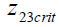for the problem.

STEP 9. The critical distance can be calculated by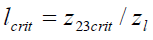(30)

Where zl is the impedance in pu per meter of the faulted line 2-4.

Evaluation of the critical impedance in real six bus network

The simple six bus system which is used in the solution of many power system analysis problems  was used for evaluating the developed procedure in section 4 to calculate the critical distance in meshed faulted power system. The single line diagram of the 6-bus power system network is shown in Figure 4 . The base line-to-line voltage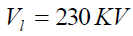, and the base apparent power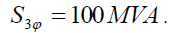The transient impedances of the generators are given in PU in Table 1.

A balanced three-phase fault is assumed at line 4-5, and so node 7 will be added at the fault location. The line data containing the series resistance and reactance in per unit, and the total capacitance in per unit susceptance are tabulated below in Table 2.

The prefault load, voltage magnitude and generation schedule for the regulated buses are tabulated in Table. 3. Bus 1 is a slack bus, whose voltage is specified as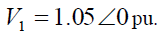The Ybus matrix and positive sequence matrix were evaluated initially while considering the fault is middle of the line 4-5, so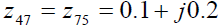pu. The load flow solution was obtained at prefault condition and the results are tabulated in Table. 4.

The fault analysis was performed when the three-phase fault at node 7 is assumed initially in the middle of line 4-5, and the values for bus voltages during the three- phase fault are tabulated in Table. 5. The fault impedance is considered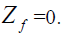Table. 5. Bus voltages during the three-phase fault at node 7 when assumed initially in the middle of line 4-5.

The proposed procedure in section 4 is applied to obtain the critical impedance along the faulted line that will cause a voltage dip in bus 4 equal to 0.4 pu, V4 =0.4 pu, and the following solution for the critical impedance is obtained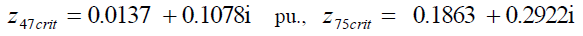pu.

The critical distance can be calculated by dividing the critical impedance by the impedance per meter of the line 4-5. The values for bus voltages during the three- phase fault after the calculation of the critical impedance are tabulated in Table. 6.

Table. 6. Bus voltages during the three-phase fault at node 7 after calculation of the critical impedance that will cause a voltage dip at bus 4 equal to 0.4 pu, V4=0.4 pu.

If the problem was solved to find the critical impedance along the faulted line that will cause a voltage dip equal to 0.5 pu at the other end of the line,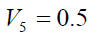pu, the following solution was obtained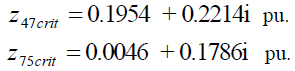The values for bus voltages during the three- phase fault after the calculation of the critical impedance in this case are tabulated in Table. 7.

Table. 7. Bus voltages during the three-phase fault at node 7 after calculation of the critical impedance that will cause a voltage dip at bus 5 equal to 0.5 pu, V5=0.5 pu

#### Calculation of critical distance by neglecting the transmission lines resistances

The critical impedance obtained in the example in section 5 is a complex quantity, and so the critical distance calculated by dividing the critical impedance by the impedance per meter of the line 4-5 will be also complex quantity, which is not acceptable. The best way to overcome the problem is to ignore the resistances of transmission lines.

If the transmission lines resistances in the 6-bus system are ignored, and the proposed procedure in section 4 is applied to calculate the critical impedance along the faulted line 4-5 that will cause a voltage dip equal to 0.4 in bus 4, V4 =0.4 pu, the solution for critical impedance will be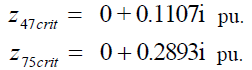The values for bus voltages during the three-phase fault after the calculation of the critical impedance are tabulated in Table. 8.

Table. 8. Bus voltages during the three-phase fault at node 7 after calculation of the critical impedance that will cause a voltage dip at bus 4 equal to 0.4 pu.

Table. 9. Bus voltages during the three-phase fault at node 7 after calculation of the critical impedance that will cause a voltage dip at bus 5 equal to 0.5 pu

The critical distance can be calculated by dividing the critical impedance by the impedance per meter of the line 4-5. If the impedance per Km of the line is j1 pu/Km, the critcal distance will be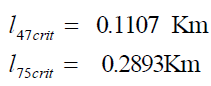If the desired voltage dip at bus 4 is varied to be from 0 pu to 1 pu, Figure 5 shows the critical distance solution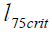and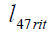for the various desired voltage dip magnitudes at bus 4.

Fig. 5. The critical distance l47rit and l75crit for various desired voltage dip magnitudes at bus 4 (0 PU – 1 PU).

If the desired fault voltage at bus 5 is varied to be from 0 pu to 1 pu, Figure 6 shows the critical distance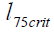and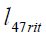for the various desired voltage dip magnitudes at bus 5.

Fig. 6. The critical distances l47rit and l75crit for various desired voltage dip magnitudes at bus 5 (0 PU – 1 PU).

#### Discussion

The critical distance method which is based on the concept of potential divider, was correctly and easily applicable to a radial network, however non of the recent resecrches could solve the problem for meshed power system and the problem was highlighted to have a lot of complications.

The paper developed an analytical method to calculate the critical distance of a three-phase fault on transmission line that will cause certain voltage dip at a bus in meshed power system. The method is based in Gauss-Seidel iteration.

The solution started first by solving the fault analysis problem assuming the fault in the middle of the line. Then all generator buses and the faulted bus are considered as slack buses with the their voltage magnitudes specified to the values obtained from the fault analysis solution. The bus that must have the desired voltage dip magnitude is considered also as a slack bus. Then, the Gauss-Seidel iterative method was used to find the solution for the unknown bus voltages and the falulted line impedance that causes the desired voltage dip. The previous process is repeated many times until the fault analysis solution gives the desired voltage dip at the selected bus and so the critical impedance solution is obtained.

The proposed method is tested on 6-bus transmission network in Figure 4 when the three-phase fault is assumed at node 7 in line 4-5. The critical impedance obtained is a complex quantity, so the critical distance calculated by dividing the critical impedance by the impedance per meter of the line will be also complex quantity which is not acceptable. It was necessary to ignore the resistances of transmission lines in order to obtain a real solution for critical distance. The developed procedure is applied to obtain the critical impedance along the faulted line between bus 4 and 5 that will cause a voltage dip in bus 4 equal to 0.4 pu,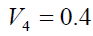pu, the solution for critical impedance is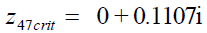pu, and so the critical distance when the impedance per Km of the line is j1 pu will be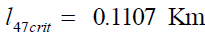Then the problem solved to find the critical impedance along the faulted line 4-5 that will cause a voltage dip equal to 0.5 pu at the other end of the line,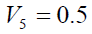pu, the solution for critical impedance is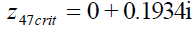pu, and so the critical distance when the impedance per Km of the line is j1 pu will be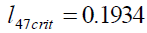Km. Plots of the critical distance for various desired voltage dip magnitudes at bus 4 and bus 5 have been developed as shown in Figure 5 and Figure 6, and the results showed significant advantages of the proposed method. However, it is noted that, it is possible to obtain the critical impedance solution assuming the desired voltage dip should be at any of the nodes that the faulted line was connected to, i.e. nodes 4 and 5 in the example, while the solution will not converge if the desired voltage dip assumed on any other bus in the system.

#### Conclusion

Faults studies form an important part of power system analysis. The problem of fault location has been studied deeply for transmission lines due its importance in the power system. Different methods for sags prediction have been developed. The most used are “critical distance” and “fault positions”. The critical distance method is based on the concept of potential divider, which is correctly and easily applicable to a radial network. The extension of this method to large meshed networks has been discussed but yet non of the existing researches could provide proper solution for thr problem. In this paper, an elegant, analytical method is developed to calculate the critical distance of a three-phase fault on transmission line that will cause certain voltage dip at a bus in meshed power system.

The paper started by giving a brief description to the solution of the balanced three-phase to ground short circuit fault problem. The problem consists of determining bus voltages and line currents during the three-phase fault when the fault location is known. The power flow solution using Gauss-Seidel is explained also. Then the proposed approach for the calculation of critical distance in meshed power system is introduced. The main objective was to calculate the critical distance of a three-phase fault along a transmission line that will cause certain voltage dip at selected bus in meshed power system.

The proposed method is tested on 6-bus transmission network. The critical impedance obtained is a complex quantity, so the critical distance calculated by dividing the critical impedance by the impedance per meter of the line will be also complex quantity which is not acceptable. It was necessary to ignore the resistances of transmission lines in order to obtain a real solution for critical distance. Plots of the critical distance for various desired voltage dip magnitudes at certain buses are obtained, and the results showed significant advantages of the proposed method. But it is noted that it is possible to obtain the critical impedance solution assuming the desired voltage dip should be at any of the nodes that the faulted line was connected to.

#### References

Select your language of interest to view the total content in your interested language

### Article Usage

• Total views: 12963
• [From(publication date):
June-2012 - Nov 14, 2019]
• Breakdown by view type
• HTML page views : 9126Can't read the image? click here to refresh